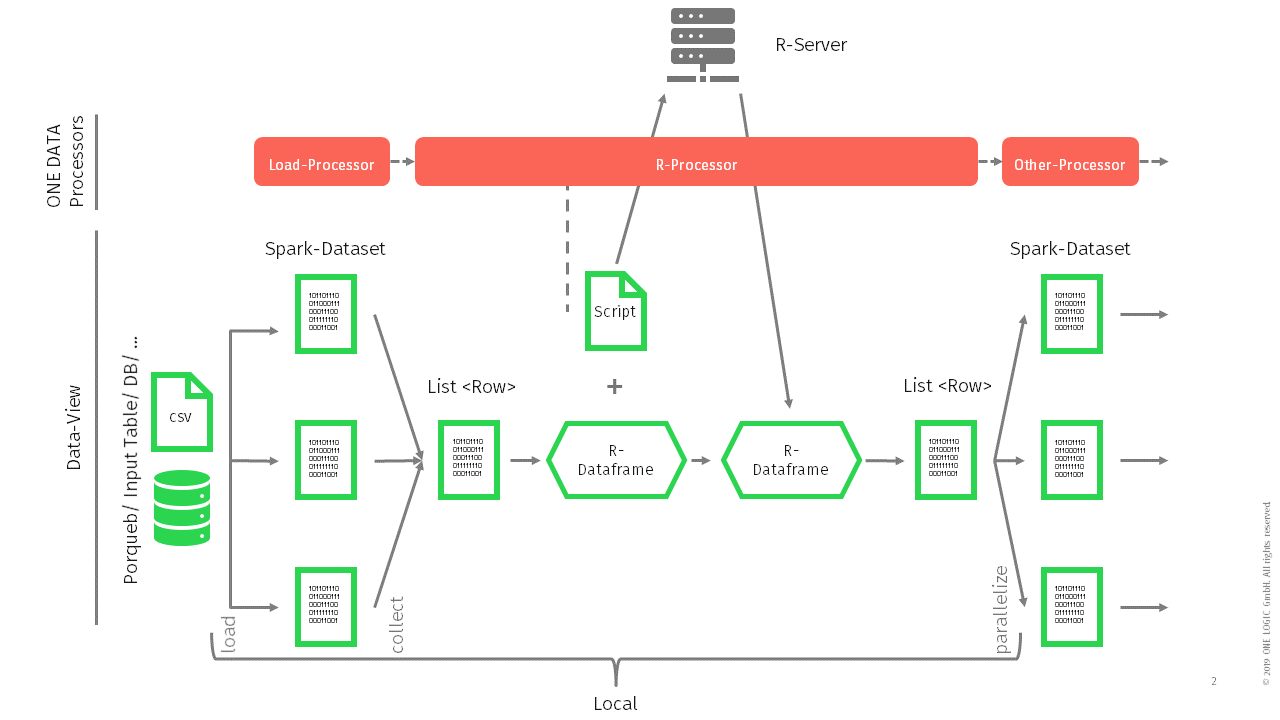# Background information - communication of ONE DATA and R-Server

For using R in ONE DATA it is usually necessary to have a separate R-Server installed. The communication between R-Server and ONE DATA can be illustrated roughly as given below.In ONE DATA the data is usually given as a spark dataset (RDD) that is distributed to several nodes. As R does not operate on distributed datasets before sending the data to the R-Server, all the data is collected into one single list which is then converted into a R-Dataframe.

This R-Dataframe along with the entered R-Script in the R-Processor is sent to the R-Server for execution. This is also one of the reasons why using R in ONE DATA might take longer than actually using it locally on the computer as first of all the data has to be converted.

When converting the dataset, currently all strings and datetimes in ONE DATA are treated as strings (using REXPString.java) and all numeric values are treated as double (using REXPDouble.java). Therefore for example integer values in ONE DATA are originally treated as double.

The R-Script is then executed on the R-Dataframe. After the execution, a R-Dataframe will be returned to ONE DATA and converted to a Spark RDD again.

The basic implementation for the communication between ONE DATA and R-Server is the following artifact:

https://mvnrepository.com/artifact/org.rosuda.REngine/Rserve/1.8.1

https://github.com/s-u/REngine

# Installation and usage of packages

## Installation of R-Folder in Directory

To install and load R packages within the R Processor prior a dedicated folder has to be installed on the server where ONE DATA and RServe are running. This folder has to be mentioned in further commands, e.g. when installing or loading R packages within the R Processor (see below).

## Installing R-Packages in the R-Script

When using the "install.packages()" command in R to install packages from CRAN-like repositories or from local files, some parameters must be specified using R with ONE DATA:

• lib: character vector giving the library directories where to install the packages, this needs to be a prior dedicated folder that was installed on the server where ONE DATA and RServe are running. (e.g.: "/home/onelogic/rpackages")
• repos: character vector, the base URL(s) of the repositories to use (e.g., the URL of a CRAN mirror such as "https://cran.cnr.berkeley.edu/")
• dependencies: logical indicating whether to also install uninstalled packages which these packages depend on/link to/import/suggest (and so on recursively), e.g.: TRUE, FALSE (usually set to TRUE)

When loading a package it is required to specify the location where this package was installed:

Example:

`library(forecast, lib.loc = "/home/onelogic/rpackages")`

# Using R in ONE DATA - good to know

1) Do not select columns in the R-Script by their position

• Don't:
`data[,(1:3)]`

• Do:
`data[,("columnname1","columnname2","columnname3"]`

Explanation:

ONE DATA and Spark do not have a fixed column order. So when collecting the distributed data before sending it to RServe there is no guarantee that the columns will be in the same order each time. This might lead to a random selection of columns when columns are selected by position in the R-Script. Therefore, with each execution the script might give different results.

2) Do not use integers in the output R data.frame

3) Debugging R-Script in ONE DATA

If it is necessary and no local debugging is possible, it might be helpful to:

• Use the "write.csv" command and save in between results to some file directory on the server the R-Server has access to. For example:
`write.csv(data, file = 'usr/lib64/R/bin/data.csv')`

`read.csv(file = '/usr/lib64/R/bin/data.csv', sep=',', header =T)`

• The R-command "capture.output" might be very useful as it helps to get output that can for example only be seen on the R-Console or does not have a dataframe/ table format, e.g. capturing the whole output of an API request:
```lapply(buildability_response, function(x) {capture.output(x)}),
function(y){as.character(paste(y, collapse =' '))```

# Related Articles

R-Script Data Generator Processor

R-Script Single Input Processor

R-Script Dual Input Processor